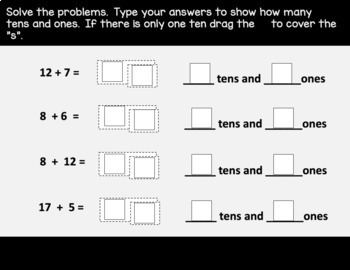# Distance Learning | Grade 1 Math Practice: Module 2 - Topic D (Eureka/EngageNY)K - 2nd
Subjects
Standards
Resource Type
Formats Included
•Google Drive™ folder
• Internet Activities#### Also included in

1. This product contains digital problem sets that are aligned to Eureka Grade 1 - Module 2.  The problems were created using Google Slides and contain moveable pieces which act as virtual math manipulatives for students.  Use these engaging practice problems to reinforce learning from your lessons eit
\$22.80
\$28.50
Save \$5.70
2. This product contains a FULL YEAR of digital problem sets that are aligned to the Eureka/Engage NY Grade 1 Math Curriculum.  The problems were created using Google Slides and contain moveable pieces which act as virtual math manipulatives for students.  Use these engaging practice problems to reinf
\$102.55
\$146.50
Save \$43.95

### Description

This product contains four digital problem sets that are aligned to Grade 1 Eureka Math or Engage NY Module 2 - Topic D.  The problems were created using Google Slides and contain moveable pieces which act as virtual math manipulatives for students.  Use these engaging practice problems to reinforce learning from your lessons either through virtual learning or through a technology center.

This problem set was designed to meet the needs of all learners.  Slides were designed using simple colors and without distracting clipart to ensure that students with learning differences and those who receive special education services are able to focus.

This product includes:

• Lesson 26 Practice - 4 problems ask students to drag and drop to cover ten, type number, and determine how many tens/ones; 4 problems ask students to show the total tens and ones with Hide Zero card and type how many tens/ones.
• Lesson 27 Practice - 8 problems ask students to solve the problem, type to show how many tens/ones, and drag and drop to cover the s if there is one ten; 3 problems ask students to drag and drop to make a drawing to match a story, show tens and ones and drag and drop to cross out "s" if there is one ten.
• Lesson 28 Practice - 8 problems ask students to solve the problem and show their solution in two steps; 3 problems ask students to solve a word problem and type a statement.
• Lesson 29 Practice - 4 problems ask students to solve the problem and show their solution in two steps; 3 problems ask students to solve then type two number sentences, and a statement to show how they took from ten.

Check out some of my other products for special education teachers:

Grade 1 Math IEP Goal Bank

Grade K-5 Math IEP Goal Bank

Grade 1 Distance Learning Math Practice Bundle: Module 1

Grade 1 Distance Learning Math Practice Module 2 - Topic A

Grade 1 Distance Learning Math Practice Module 2 - Topic B

Grade 1 Distance Learning Math Practice Module 2 - Topic C

Disclaimer: Please note that these are not the same exact problems from the Eureka curriculum. I am not associated with Eureka, Engage NY, Great Minds, etc. These are meant to be used as additional or alternative practice of the skills that have been covered in each lesson. You are paying for my approach to making the learning from each lesson accessible to students in a virtual learning environment.

Total Pages
N/A
Teaching Duration
N/A
Report this Resource to TpT
Reported resources will be reviewed by our team. Report this resource to let us know if this resource violates TpT’s content guidelines.

### Standards

to see state-specific standards (only available in the US).
Add and subtract within 20, demonstrating fluency for addition and subtraction within 10. Use strategies such as counting on; making ten (e.g., 8 + 6 = 8 + 2 + 4 = 10 + 4 = 14); decomposing a number leading to a ten (e.g., 13 - 4 = 13 - 3 - 1 = 10 - 1 = 9); using the relationship between addition and subtraction (e.g., knowing that 8 + 4 = 12, one knows 12 - 8 = 4); and creating equivalent but easier or known sums (e.g., adding 6 + 7 by creating the known equivalent 6 + 6 + 1 = 12 + 1 = 13).
Use addition and subtraction within 20 to solve word problems involving situations of adding to, taking from, putting together, taking apart, and comparing, with unknowns in all positions, e.g., by using objects, drawings, and equations with a symbol for the unknown number to represent the problem.
The numbers from 11 to 19 are composed of a ten and one, two, three, four, five, six, seven, eight, or nine ones.
10 can be thought of as a bundle of ten ones - called a “ten.”